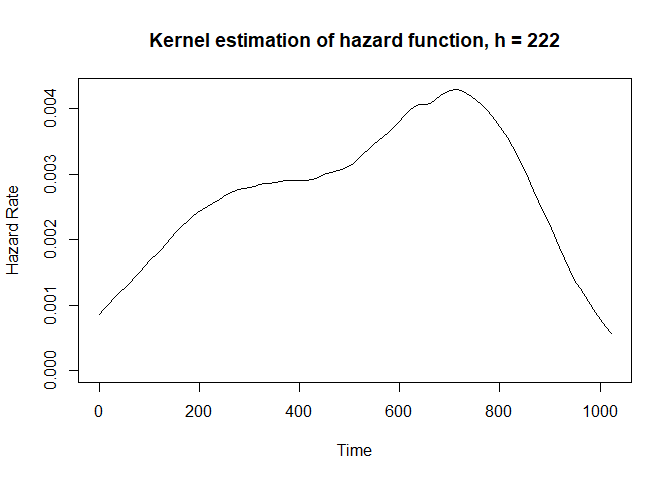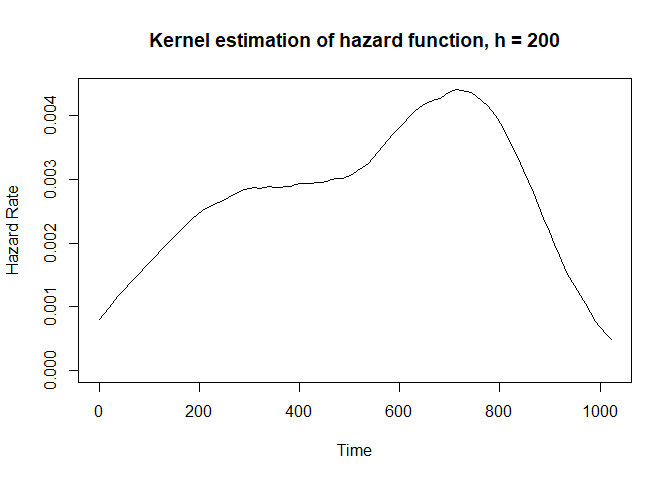# kernhaz

The goal of kernhaz is to estimate the unconditional and the conditional hazard function for right-censored data. The package includes two methods of the bandwidth selection - crossvalidation and maximum likelihood method.

## Installation

You can install the released version of kernhaz from CRAN with:

``install.packages("kernhaz")``

## Example

The unconditional hazard function, the bandwidth is selected using the crossvalidation method:

``````library(survival)
fit1<-khazard(times = lung\$time,delta = lung\$status-1)
plot(fit1)``````The unconditional hazard function, four bandwidths are considered:

``````library(survival)
fit2<-khazard(times = lung\$time,delta = lung\$status-1,h=c(100,150,200,250), value="both")
plot(fit2,h=200)``````The conditional hazard function, bandwidths are selected using the crossvalidation method:

``````library(survival)
fit3<-khazardcond(times = lung\$time,delta = lung\$status-1,covariate = lung\$age)
plot(fit3)``````The conditional hazard function, bandwidths are user’s choice:

``````library(survival)
fit4<-khazardcond(times = lung\$time,delta = lung\$status-1,covariate = lung\$age,h=c(200,20))
plot(fit4)``````